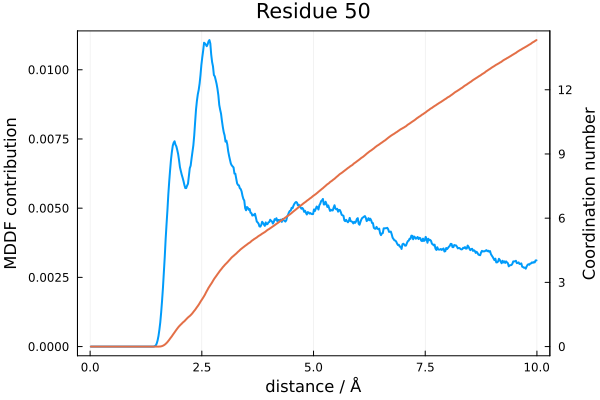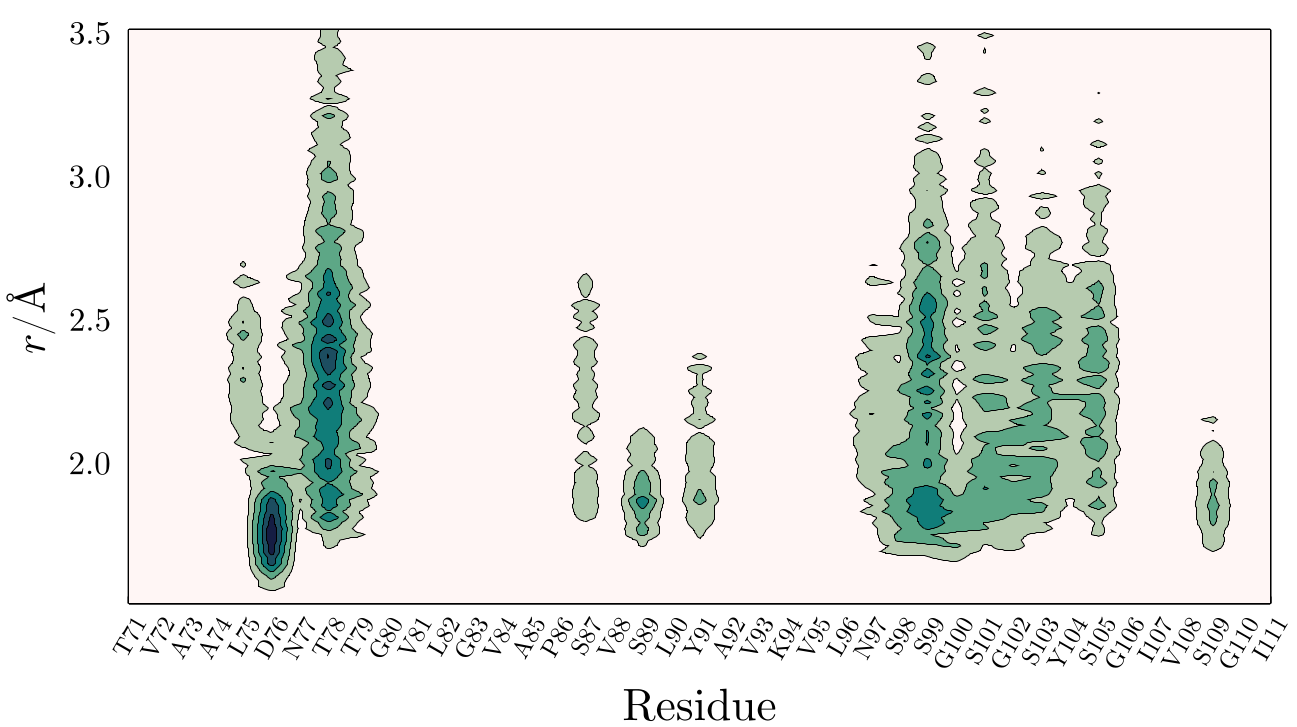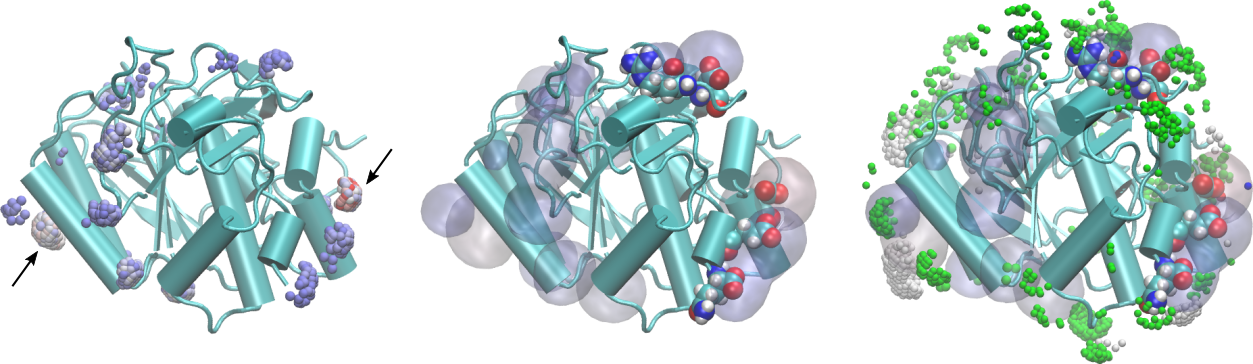# Tools

A set of examples of analyses that can be performed with ComplexMixtures is given in this site. A brief the description of the possible results is provided here.

Some tools are provided to analyze the results:

## Coordination numbers

The function

coordination_number(R::Result, group_contributions::Vector{Float64})

computes the coordination number of a given group of atoms from the solute or solvent atomic contributions to the MDDF. Here, R is the result of the mddf calculation, and group_contributions is the output of the contributions function for the desired set of atoms.

If no group is defined, the coordination number of the complete solute is returned, which is equivalent to the R.coordination_number field of the Result data structure:

coordination_number(R::Result) == R.coordination_number
Note

There are some systems for which the normalization of the distributions is not necessary or possible. It is still possible to compute the coordination numbers, by running, instead of mddf, the coordination_number function:

coordination_number(trajectory::Trajectory, options::Options)

This call will return Result data structure but with all fields requiring normalization with zeros. In summary, this result data structure can be used to compute the coordination numbers, but not the MDDF, RDF, or KB integrals.

Compat

The use independent computation of coordination numbers was introduced in version 1.1.

### Example

In the following example we compute the coordination number of the atoms of residue 50 (which belongs to the solute - a protein) with the solvent atoms of TMAO, as a function of the distance. The plot produced will show side by side the residue contribution to the MDDF and the corresponding coordination number.

using ComplexMixtures, PDBTools
using Plots, EasyFit
solute = Selection(PDBTools.select(pdb, "protein"), nmols=1)
residue50 = PDBTools.select(pdb, "residue 50")
# Compute the group contribution to the MDDF
residue50_contribution = contributions(solute, R.solute_atom, residue50)
# Now compute the coordination number
residue50_coordination = coordination_number(R, residue50_contribution)
# Plot with twin y-axis
plot(R.d, movavg(residue50_contribution,n=10).x,
xaxis="distance / Å",
yaxis="MDDF contribution",
linewidth=2, label=nothing, color=1
)
plot!(twinx(),R.d, residue50_coordination,
yaxis="Coordination number",
linewidth=2, label=nothing, color=2
)
plot!(title="Residue 50", framestyle=:box, subplot=1)

With appropriate input data, this code produces:## Computing a 2D density map around a macromolecule

One nice way to visualize the accumulation or depletion of a solvent around a macromolecule (a protein, for example), is to obtain a 2D map of the density as a function of the distance from its surface. For example, in the figure below the density of a solute (here, Glycerol), in the neighborhood of a protein is shown:Here, one can see that Glycerol accumulates on Asp76 and on the proximity of hydrogen-bonding residues (Serine residues mostly). This figure was obtained by extracting from atomic contributions of the protein the contribution of each residue to the MDDF. Using PDBTools, this can be done with, for example:

residues = collect(eachresidue(protein))
residue_contributions = zeros(length(R.d),length(residues))
for (i,residue) in pairs(residues)
c = contributions(solute,R.solute_atom,residue)
residue_contributions[:,i] .= c
end

The above produces a matrix with a number of columns equal to the number of residues and a number of rows equal to the number of MDDF points. That matrix can be plotted as a contour map with adequate plotting software. A complete running example is provided here, producing the figure above.

## Computing a 3D density map around a macromolecule

Three-dimensional representations of the distribution functions can also be obtained from the MDDF results. These 3D representations are obtained from the fact that the MDDFs can be decomposed into the contributions of each solute atom, and that each point in space is closest to a single solute atom as well. Thus, each point in space can be associated to one solute atom, and the contribution of that atom to the MDDF at the corresponding distance can be obtained.

For example, the distribution function of a hydrogen-bonding liquid solvating a protein will display a characteristic peak at about 1.8Å. The MDDF at that distance can be decomposed into the contributions of all atoms of the protein which were found to form hydrogen bonds to the solvent. A 3D representation of these contributions can be obtained by computing, around a static protein (solute) structure, which are the regions in space which are closer to each atom of the protein. The position in space is then marked with the atom of the protein to which that region "belongs" and with the contribution of that atom to the MDDF at each distance within that region. A special function to compute this 3D distribution is provided here: grid3D.

This is better illustrated by a graphical representation. In the figure below we see a 3D representation of the MDDF of Glycerol around a protein, computed from a simulation of this protein in a mixture of water and Glycerol. A complete set of files and a script to reproduce this example is available here.In the figure on the left, the points in space around the protein are selected with the following properties: distance from the protein smaller than 2.0Å and relative contribution to the MDDF at the corresponding distance of at least 10% of the maximum contribution. Thus, we are selecting the regions of the protein corresponding to the most stable hydrogen-bonding interactions. The color of the points is the contribution to the MDDF, from blue to red. Thus, the most reddish-points corresponds to the regions where the most stable hydrogen bonds were formed. We have marked two regions here, on opposite sides of the protein, with arrows.

Clicking on those points we obtain which are the atoms of the protein contributing to the MDDF at that region. In particular, the arrow on the right points to the strongest red region, which corresponds to an Aspartic acid. These residues are shown explicitly under the density (represented as a transparent surface) on the figure in the center.

The figure on the right displays, overlapped with the hydrogen-bonding residues, the most important contributions to the second peak of the distribution, corresponding to distances from the protein between 2.0 and 3.5Å. Notably, the regions involved are different from the ones forming hydrogen bonds, indicating that non-specific interactions with the protein (and not a second solvation shell) are responsible for the second peak.

An example input file which produces the files required for producing these images is:

using ComplexMixtures, PDBTools

# PDB file of the system simulated

# Load results of a ComplexMixtures run

# Inform which is the solute
protein = select(pdb,"protein")
solute = Selection(protein,nmols=1)

# Compute the 3D density grid and output it to the PDB file
grid = grid3D(
solute=solute,
solute_atoms=protein,
mddf_result=R,
output_file="grid.pdb"
)

The call to grid3D in the last command will write an output a PDB file with the grid points, which loaded in a visualization software side-by-side with the protein structure, allows the production of the images shown. The grid.pdb file contains a regular PDB format, but the atoms are grid points. The identity of the atoms correspond to the identity of the protein atom contributing to the MDDF at that point (the closest protein atom). The temperature-factor column (beta) contains the relative contribution of that atom to the MDDF at the corresponding distance, and the occupancy field contains the distance itself.

The output grid variable contains the same information of the PDB file, which can be analyzed with the tools of PDBTools if the user wants to.

The distributions returned by the mddf function (the mddf and rdf vectors), are normalized by the random reference state or using a site count based on the numerical integration of the volume corresponding to each minimum-distance to the solute.

If, however, the solute is defined by a single atom (as the oxygen atom of water, for example), the numerical integration of the volume can be replaced by a simple analytical spherical shell volume, reducing noise. The ComplexMixtures.gr function returns the radial distribution function and the KB integral computed from the results, using this volume estimate:

g, kb = ComplexMixtures.gr(R)

By default, the single-reference count (rdf_count) of the Result structure will be used to compute the radial distribution function. The function can be called with explicit control of all input parameters:

g, kb = ComplexMixtures.gr(r,count,density,binstep)

where:

ParameterDefinitionResult structure output data to provide
rVector of distancesThe d vector
countNumber of site counts at each rThe rdf or mddf vectors
densityBulk densityThe density.solvent_bulk or density.solvent densities.
binstepThe histogram stepThe options.binstep

Example:

...
R = mddf(trajectory,options)
g, kb = ComplexMixtures.gr(R.d,R.rdf_count,R.density.solvent_bulk,R.options.binstep)

## Overview of the solvent and solute properties

The output to the REPL of the Result structure provides an overview of the properties of the solution. The data can be retrieved into a data structure using the overview function. Examples:

...
julia> results = mddf(trajectory)

julia> results

-------------------------------------------------------------------------------

MDDF Overview:

Solvent properties:
-------------------

Simulation concentration: 1.5209006318095133 mol L⁻¹
Molar volume: 657.5051512801567 cm³ mol⁻¹

Concentration in bulk: 1.4918842545752287 mol L⁻¹
Molar volume in bulk: 670.2932864484995 cm³ mol⁻¹

Solute properties:
------------------

Simulation Concentration: 1.5209006318095133 mol L⁻¹
Estimated solute partial molar volume: 657.5051512801567 cm³ mol⁻¹

Using dbulk = 20.0Å:
Molar volume of the solute domain: 30292.570006549242 cm³ mol⁻¹

Auto-correlation: true

Trajectory files and weights:
./vinicius.xtc - w = 1.0

Long range MDDF mean (expected 1.0): 1.1090804621839963 +/- 0.04298849642932878
Long range RDF mean (expected 1.0): 1.15912932236198 +/- 0.05735018864444404

-------------------------------------------------------------------------------

In this case, since solute and solvent are equivalent and the system is homogeneous, the molar volumes and concentrations are similar. This is not the case if the molecules are different or if the solute is at infinite dilution (in which case the bulk solvent density might be different from the solvent density in the simulation).

To retrieve the data of the overview structure use, for example:

julia> overview = overview(results);

julia> overview.solute_molar_volume
657.5051512801567